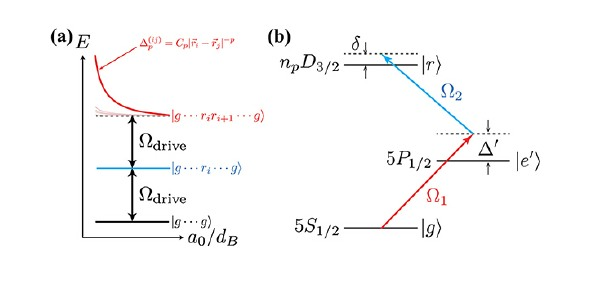What is the frequency of a photon absorbed when the atom undergoes a - ProProfs DiscussTopicsMoreProductsMore+ Ask Question

# What is the frequency of a photon absorbed when the atom undergoes a transition from n1=2 to n2=4. (R=1.096776x107m-1; c=3.0x108 m/s)?Change Image    Delete

A. 2.056x10^6 s^-1
B. 2.742x10^6 s^-1
C. 6.165x10^14 s^-1
D. 8.226x10^14 s^-1
E. >10^15 s^-1

This question is part of electron configuration Test
Asked by Bbrocker, Last updated: Feb 16, 2020

###Request 0FollowShareAnswer AnonymouslyAnswer LaterCopy Link#### Matz Lewis Clark

Traveler and writer by profession.

Matz Lewis Clark, College student, Graduation, Orlando

The correct answer to this question is C, 6.165x10^14 s^-1. This type of question could be found on a chemistry test, as it is related to electron configuration, which is when electrons are distributed throughout an atom. This atom can either be neutral or ionic. It also involves the distribution of molecules in orbitals.

Pauli-Exclusion Principle, Hund's Rule, and Aufbau's Principle are three of the rules to solve electron configuration. Aufbau's Principle found that electrons take up the orbital in the order of energy increasements. Hund's Rule states that electrons must be emptied from an orbital before space can be occupied again. Pauli-Exclusion Principle states the electrons can have a unique set of four numbers.#### l.Steven

L.Steven

The Rydberg formula is an ingenious mathematical revolution in atomic physics, which allows you to describe different wavelengths of the spectral lines of different chemical elements. As he was trying to arrive at his formula, he found the Balmer’s formula, which Balmer created for hydrogen atoms.

By examining all the different lines in the spectrum of hydrogen atoms, he devised an empirical model, which could explain and predict the pattern of emission. The formula was 6.165x10^14 s^-1. It is amazing how one set of information can be used to build upon. The original Balmer’s formula was discovered in 885. The Rydberg formula came into existence, 3 years later and is a generalization of this formula.#### John Smith

John Smith6.165x10^14 s^-1Search for Google imagesSelect a recommended image
Upload from your computerCancelSearch for Google imagesSelect a recommended image
Upload from your computerCancelSearch for Google imagesSelect a recommended image
Upload from your computerCancel